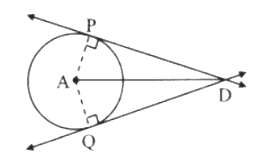# Proof of tangent segment theorem.

Statement: The segments of the tangent drawn from a point outside (external point) to a circle are congruent.

Proof:

Construction: Draw two segments AP and AQ.According to the figure, A is the centre of the circle. The tangents drawn through point D from outside the circle touches the circle at the points P and Q.

seg PA ≅ [segQA] [Radii of the same circle]

∠APD = ∠AQD = 90° [Tangent theorem]

∴ seg DP = seg DQ [congruent sides of congruent triangles](0)(0)